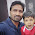Electrical Objective Questions with Answers GK - Set 01 - ObjectiveBooks

# G.K. Practice Test: Question Set - 01

1. Which of the following parameter will be more for 16 gauge copper wire in comparison to 14 gauge copper wire?
(A) Cost
(B) Strength
(C) Resistance
(D) Weight

2. The length of wire having resistance of 1 ohm/m in a heater rated at 1000 W and 250 V will be
(A) 250 m
(B) 125 m
(C) 62.5 m
(D) 500 m

3. Power dissipated in a pure capacitor is
(A) Minimum
(B) Maximum
(C) Infinite
(D) Zero

4. The size of the feeder is determined primarily by
(A) The current it is required to carry
(B) The percentage variation of voltage in the feeder
(C) The voltage across the feeder
(D) The distance over which the transmission is made

5. Non-conductors whose polarization is caused by an electric field are known as
(A) Dielectric
(B) Super-conductors
(C) Semi-conductors
(D) Insulators

6. If the diameter of a conductor is double, then its resistance will be reduced to
(A) Half
(B) One-fourth
(C) One-eight
(D) One-sixteenth

7. One of difficulties encountered with super-conductors is
(A) The materials become highly magnetic
(B) Strength is reduced
(C) Resistance is increased
(D) Maintenance of low temperature

8. The inductance of a coil can be increased by
(A) Decreasing number of turns
(B) Increasing core length
(C) Using core material of high relative permeability
(D) All of the above

9. The resistance of a lamp rated at 240 V and 60 watts equal to
(A) 960 ohms
(B) 120 ohms
(C) 240 ohms
(D) 480 ohms

10. Two resistances of 100 ohms and 0 ohm are connected in parallel. The overall resistance will be
(A) 100 ohms
(B) 50 ohms
(C) Zero ohm
(D) Any one of the above

Show and hide multiple DIV using JavaScript View All Answers

Blogger Comment
1.1.